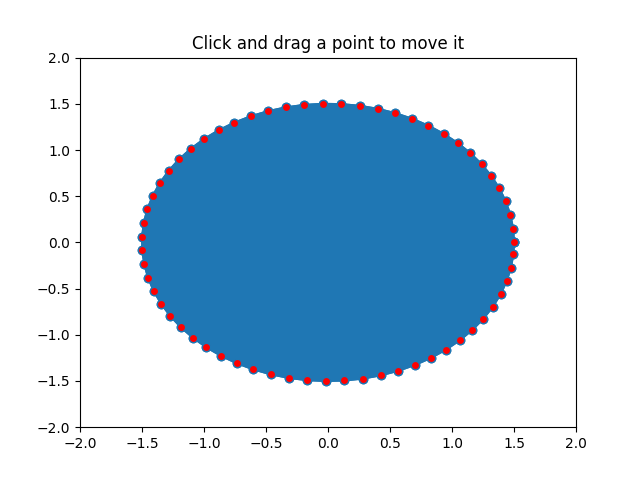# Poly Editor¶

This is an example to show how to build cross-GUI applications using Matplotlib event handling to interact with objects on the canvas.import numpy as np
from matplotlib.lines import Line2D
from matplotlib.artist import Artist

def dist(x, y):
"""
Return the distance between two points.
"""
d = x - y
return np.sqrt(np.dot(d, d))

def dist_point_to_segment(p, s0, s1):
"""
Get the distance of a point to a segment.
*p*, *s0*, *s1* are *xy* sequences
This algorithm from
http://geomalgorithms.com/a02-_lines.html
"""
v = s1 - s0
w = p - s0
c1 = np.dot(w, v)
if c1 <= 0:
return dist(p, s0)
c2 = np.dot(v, v)
if c2 <= c1:
return dist(p, s1)
b = c1 / c2
pb = s0 + b * v
return dist(p, pb)

class PolygonInteractor:
"""
A polygon editor.

Key-bindings

't' toggle vertex markers on and off.  When vertex markers are on,
you can move them, delete them

'd' delete the vertex under point

'i' insert a vertex at point.  You must be within epsilon of the
line connecting two existing vertices

"""

showverts = True
epsilon = 5  # max pixel distance to count as a vertex hit

def __init__(self, ax, poly):
if poly.figure is None:
raise RuntimeError('You must first add the polygon to a figure '
'or canvas before defining the interactor')
self.ax = ax
canvas = poly.figure.canvas
self.poly = poly

x, y = zip(*self.poly.xy)
self.line = Line2D(x, y,
marker='o', markerfacecolor='r',
animated=True)

self._ind = None  # the active vert

canvas.mpl_connect('draw_event', self.on_draw)
canvas.mpl_connect('button_press_event', self.on_button_press)
canvas.mpl_connect('key_press_event', self.on_key_press)
canvas.mpl_connect('button_release_event', self.on_button_release)
canvas.mpl_connect('motion_notify_event', self.on_mouse_move)
self.canvas = canvas

def on_draw(self, event):
self.background = self.canvas.copy_from_bbox(self.ax.bbox)
self.ax.draw_artist(self.poly)
self.ax.draw_artist(self.line)
# do not need to blit here, this will fire before the screen is
# updated

def poly_changed(self, poly):
"""This method is called whenever the pathpatch object is called."""
# only copy the artist props to the line (except visibility)
vis = self.line.get_visible()
Artist.update_from(self.line, poly)
self.line.set_visible(vis)  # don't use the poly visibility state

def get_ind_under_point(self, event):
"""
Return the index of the point closest to the event position or *None*
if no point is within self.epsilon to the event position.
"""
# display coords
xy = np.asarray(self.poly.xy)
xyt = self.poly.get_transform().transform(xy)
xt, yt = xyt[:, 0], xyt[:, 1]
d = np.hypot(xt - event.x, yt - event.y)
indseq, = np.nonzero(d == d.min())
ind = indseq

if d[ind] >= self.epsilon:
ind = None

return ind

def on_button_press(self, event):
"""Callback for mouse button presses."""
if not self.showverts:
return
if event.inaxes is None:
return
if event.button != 1:
return
self._ind = self.get_ind_under_point(event)

def on_button_release(self, event):
"""Callback for mouse button releases."""
if not self.showverts:
return
if event.button != 1:
return
self._ind = None

def on_key_press(self, event):
"""Callback for key presses."""
if not event.inaxes:
return
if event.key == 't':
self.showverts = not self.showverts
self.line.set_visible(self.showverts)
if not self.showverts:
self._ind = None
elif event.key == 'd':
ind = self.get_ind_under_point(event)
if ind is not None:
self.poly.xy = np.delete(self.poly.xy,
ind, axis=0)
self.line.set_data(zip(*self.poly.xy))
elif event.key == 'i':
xys = self.poly.get_transform().transform(self.poly.xy)
p = event.x, event.y  # display coords
for i in range(len(xys) - 1):
s0 = xys[i]
s1 = xys[i + 1]
d = dist_point_to_segment(p, s0, s1)
if d <= self.epsilon:
self.poly.xy = np.insert(
self.poly.xy, i+1,
[event.xdata, event.ydata],
axis=0)
self.line.set_data(zip(*self.poly.xy))
break
if self.line.stale:
self.canvas.draw_idle()

def on_mouse_move(self, event):
"""Callback for mouse movements."""
if not self.showverts:
return
if self._ind is None:
return
if event.inaxes is None:
return
if event.button != 1:
return
x, y = event.xdata, event.ydata

self.poly.xy[self._ind] = x, y
if self._ind == 0:
self.poly.xy[-1] = x, y
elif self._ind == len(self.poly.xy) - 1:
self.poly.xy = x, y
self.line.set_data(zip(*self.poly.xy))

self.canvas.restore_region(self.background)
self.ax.draw_artist(self.poly)
self.ax.draw_artist(self.line)
self.canvas.blit(self.ax.bbox)

if __name__ == '__main__':
import matplotlib.pyplot as plt
from matplotlib.patches import Polygon

theta = np.arange(0, 2*np.pi, 0.1)
r = 1.5

xs = r * np.cos(theta)
ys = r * np.sin(theta)

poly = Polygon(np.column_stack([xs, ys]), animated=True)

fig, ax = plt.subplots()